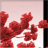# Need Help on Python Script

1308
7
10-23-2019 01:06 AMNew Contributor

Hi Folks, need help to sort my first script I have written in python (arcpy, sqlite3). Basically I am trying to update x y fields of a non-spatial sqlite3 database table (contains about 10 million records) with point geometries (SHAP@X and SHAPE@Y) values from one of the point feature layer (about 20000-30000 records), where IDs (Station_Value) match in both point feature layer and target sqlite3 database table.

The script I have written will run but take about 40 minutes to update about 20000 records. I can't really figure it out why? hope someone can help to improve it, your help in this regard will be much appreciated. Below is a sample of my script:

``````class customErr(Exception):
pass

import arcpy, sqlite3, traceback

try:
# Get parameters
inputFC = arcpy.GetParameter(0)
dbName = arcpy.GetParameterAsText(1)
tblName = arcpy.GetParameterAsText(2)
fldStn = arcpy.GetParameterAsText(3)
fldX = arcpy.GetParameterAsText(4)
fldY = arcpy.GetParameterAsText(5)

# Check FC is point type, has ID field.
inDesc = arcpy.Describe(inputFC)# Get description of FC
if inDesc.shapeType.lower() != "point":
raise customErr, "Data must be point type"
if not inDesc.hasOID:
raise customErr, "Data must have an ID field"

# Get reference to active data frame
mxd = arcpy.mapping.MapDocument("CURRENT")
df = mxd.activeDataFrame

cnGP = sqlite3.connect(dbName)

fields = ['Station_Value', 'SHAPE@X','SHAPE@Y']

with arcpy.da.SearchCursor(inputFC, fields) as cursor:

for row in (cursor):
Station_Value = row
coordX = row
coordY = row
strSQL = 'UPDATE {0} SET {3} = {5}, {4} = {6} WHERE {1} in ({2})'.format(tblName, fldStn,Station_Value,fldX,fldY,coordX,coordY)
cnGP.execute(strSQL)

cnGP.commit()
cnGP.close()

except customErr, msg:

except Exception, ErrorDesc:

finally:
try:
del inputFC, DbName, tblName, fldStn , fldX, fldY, mxd, df
del planList, inDesc, inputFC
del conn, strSQL
arcpy.Delete_management("in_memory")
except:
pass‍‍‍‍‍‍‍‍‍‍‍‍‍‍‍‍‍‍‍‍‍‍‍‍‍‍‍‍‍‍‍‍‍‍‍‍‍‍‍‍‍‍‍‍‍‍‍‍‍‍‍‍‍‍‍‍‍‍‍‍‍‍‍‍‍‍‍‍‍‍‍‍‍‍‍‍‍‍‍‍‍‍‍‍‍‍‍‍‍‍‍‍‍‍‍‍‍‍‍‍‍‍‍‍‍‍‍‍‍‍‍‍‍‍‍‍‍‍‍‍‍‍‍‍‍‍‍‍‍‍‍‍‍‍‍‍‍‍‍‍‍‍‍‍‍‍‍‍‍‍‍‍‍‍‍‍‍‍‍‍‍‍‍‍‍‍‍‍‍‍‍‍‍‍‍‍‍``````

Note: I am working in ArcGIS/Arcview Basic version 10.6.

7 RepliesbyMVP Legendary Contributor

will give line numbers so people can respond with line referencesNew Contributor

Dan, thanks, I have applied line numbers in code formatting.byMVP Esteemed Contributor

Overall, your methodology seems straightforward and sound.  I do wonder, though, why are you sorting the cursor using Python sorted?  Outside of the Python sorted consuming memory and time that may not be needed, my guess is the SQLite update is taking all the time.  I would comment out the lines where the SQLite table is updated and run the code, that will tell you how fast or slow the ArcPy portion of the code is.New Contributor

Joshua, thanks for your reply. I have removed sorting from the cursor, also I have given the code another try but result is still more or less the same. Any other idea?byMVP Frequent Contributor

Perhaps instead of UPDATE by iterating over the arcpy cursor you could instead just do a fast INSERT into a temp table in the SQL db, join that table to your destination table and run the UPDATE that way?  You'd probably have to create a StoredProcedure to run the join/update.

Seems a bit roundabout way but perhaps letting the SQL db do it's thing would be more efficient.  Sorry in advance if this is a bad idea and wasted effort (I don't have SQLLite to give it a go myself)byMVP Esteemed Contributor

Did you also try removing the SQLite execute statement to isolate whether that is the cause of the slowness?byMVP Honored Contributor

Executing 20,000 separate selections against 10 million records should take the amount of time you are seeing.  This means you have to read through the 10 million records 20,000 times.  You asked for my help based on my Turbo Charging Data Manipulation with Python Cursors and Dictionaries.  That technique solves this problem by reading the 20,000 records into memory once and then running through each of the 10 million records once updating the record from a lookup dictionary.  This eliminates 19,999 reads through the 10 million records.  So instead of iterating the 20,000 records you need to do an update iteration through the 10 million records after loading the 20,000 records in the dictionary.

I don't really know how to set up the update iteration of your 10 million records, so I can't suggest how to do that.  However, reading the 20,000 records into a dictionary and iterating through a da.updateCursor would be done with this code:

``````inputFC = arcpy.GetParameter(0)
dbName = arcpy.GetParameterAsText(1)
tblName = arcpy.GetParameterAsText(2)
fldStn = arcpy.GetParameterAsText(3)
fldX = arcpy.GetParameterAsText(4)
fldY = arcpy.GetParameterAsText(5)

# Check FC is point type, has ID field.
inDesc = arcpy.Describe(inputFC)# Get description of FC
if inDesc.shapeType.lower() != "point":
raise customErr, "Data must be point type"
if not inDesc.hasOID:
raise customErr, "Data must have an ID field"

# Get reference to active data frame
mxd = arcpy.mapping.MapDocument("CURRENT")
df = mxd.activeDataFrame

cnGP = sqlite3.connect(dbName)

fields = ['Station_Value', 'SHAPE@X','SHAPE@Y']

valueDict = {r:(r[1:]) for r in arcpy.da.SearchCursor(inputFC, fields)}‍‍

updateFC = dbName

updateFieldsList = [fldStn, fldX, fldY]

with arcpy.da.UpdateCursor(updateFC, updateFieldsList) as updateRows:
for updateRow in updateRows:
# store the Join value of the row being updated in a keyValue variable
keyValue = updateRow
# verify that the keyValue is in the Dictionary
if keyValue in valueDict:
# transfer the values stored under the keyValue from the dictionary to the updated fields.
for n in range (1,len(sourceFieldsList)):
updateRow[n] = valueDict[keyValue][n-1]
updateRows.updateRow(updateRow)

del valueDict  ‍‍‍‍‍‍‍‍‍‍‍‍‍‍‍‍‍‍‍‍‍‍‍‍‍‍‍‍‍‍‍‍‍‍‍‍‍‍‍‍‍‍‍‍‍‍‍‍‍‍‍‍‍‍‍‍‍‍‍‍‍‍‍‍‍‍‍‍‍‍‍‍‍‍‍‍‍‍‍‍‍‍‍‍‍‍‍‍‍‍‍‍‍‍‍‍‍‍‍‍‍‍‍‍‍‍‍‍‍‍‍‍‍‍‍‍‍‍‍‍‍‍‍‍‍‍‍‍‍‍‍‍‍‍‍‍‍‍‍‍‍‍‍‍‍‍‍‍‍‍‍‍‍‍‍‍‍‍‍‍‍‍‍‍‍‍‍‍‍‍‍‍‍‍‍‍‍‍‍‍‍‍‍‍‍‍‍‍‍‍‍‍‍‍‍‍‍‍‍‍‍‍‍‍‍‍‍‍‍‍‍‍‍‍‍‍‍‍‍‍‍‍‍‍‍‍‍‍‍‍‍‍‍‍``````